# Magic Sums and Products

##### Age 11 to 16

Published June 2000,July 2007,August 2007,February 2011.

The well-known magic square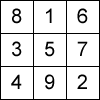has the property that the three numbers in each of the three rows, the three columns and the two diagonals all add up to the same number $15$. This number is called the magic sum of the square.

It is less well known that if you multiply the three numbers in each row together and add the three products:

$8\times 1\times 6+3\times 5\times 7+4\times 9\times 2=225$

you get the same total as when you multiply the three numbers in each column together and add the three products:

$8\times 3\times 4+1\times 5\times 7+6\times 7\times 2=225$.

This number is called the magic product of the square.

Now take the "pairwise products'' in each row and add them all up:

$(8\times 1+1\times 6+6\times 8)+(3\times 5+5\times 7+7\times 3)+ (4\times 9+9\times 2+2\times 6)=195$.

Do the same with the columns:

$(8\times 3+3\times 4+4\times 8)+(1\times 5+5\times 9+9\times 1)+ (6\times 7+7\times 2+2\times 6)=195$.

Again the results are the same! This number is called the magic pairwise product of the square.

The only other $3\times 3$ magic squares using the numbers from $1$ to $9$ are just reflections and rotations of the magic square above, such as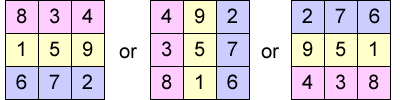These examples all have the same magic sum, magic product and magic pairwise product as the first one. However, magic squares can be formed using different sets of nine numbers. Three examples are given below.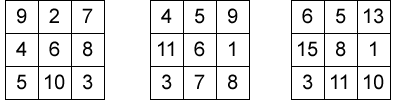Can you find other $3\times 3$ magic squares?

Of course, you could just rotate and reflect the examples above, and you could multiply every number in the square by the same constant. The new squares would still be magic, and it is not difficult to see that the magic squares you create would again have the magic product property and the magic pairwise product property.

You could also add the same number to each of the nine numbers in a magic square, and the result would clearly be a new magic square. In this case it is not at all clear that the new magic square will still have the magic product and the magic pairwise product properties.

Try to make up your own magic squares by assigning a few numbers at random to some of the cells and then filling the other cells in so that the rows, columns and diagonals add up to the same number. Each time you succeed in making a magic square, you should check that the magic product and magic pairwise product properties also work.

After having worked out a few more examples, you may notice that the magic number is always three times the middle number of the magic square. In particular, it seems that the magic number is always a multiple of $3$.

Now let's work out a systematic way of finding all $3\times 3$ magic squares.

Let the number in the middle be $x$ and the magic sum of each row, diagonal and column be $R$.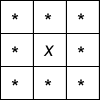Add the middle column and middle row and both diagonals giving $4R$.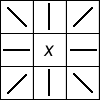This sum includes the middle number four times and all the other numbers once so it must add up to the total of all the numbers ($3R$) plus three times the middle number. So

$4R=3R+3x$

$R=3x$

This also tells us that the total of all the numbers in the square is $9x$.

Now you can make up your own magic squares. You just choose the middle number and pick two numbers in other positions and then you complete the whole magic square so each line adds up to three times the middle number.

For example, with $7$ in the middle, the row total is $21$ so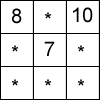must be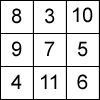If you choose the `corners' to be $x+a$ and $x+b$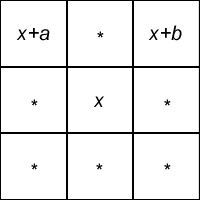then the magic square is: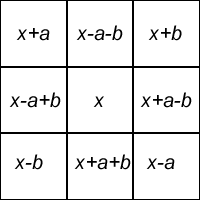It is now possible, using only elementary algebra, to check that the general $3\times 3$ magic square satisfies the magic product property and the magic pairwise product property. We leave that as an exercise for the energetic reader.

What happens with $5\times 5$ magic squares? Try the example below.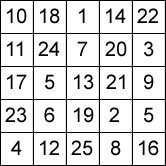What can you discover?